# 计算机编程技术之堆排序的分析与实现

 排序方法 最好时间 平均时间 最坏时间 辅助存储 选择排序 O(n^2) O(n^2) O(n^2) O(1) 插入排序 O(n) O(n^2) O(n^2) O(1) 冒泡排序 O(n) O(n^2) O(n^2) O(1) 希尔排序 O(n^1.3) O(nlogn) O(n^2) O(1) 快速排序 O(nlogn) O(nlogn) O(n^2) O(logn) 堆排序 O(nlogn) O(nlogn) O(nlogn) O(1) 归并排序 O(nlogn) O(nlogn) O(nlogn) O(n) 基数排序 O(kn) O(kn) O(kn) O(n)

1.   将序列构造成一棵完全二叉树 ；

2.   把这棵普通的完全二叉树改造成堆，便可获取最小值 ；

3.   输出最小值 ；

4.   删除根结点，继续改造剩余树成堆，便可获取次小值 ；

5.   输出次小值 ；

6.   重复改造，输出次次小值、次次次小值，直至所有结点均输出，便得到一个排序。

1．先将这些元素通过数组构造成完全二叉树。如图1所示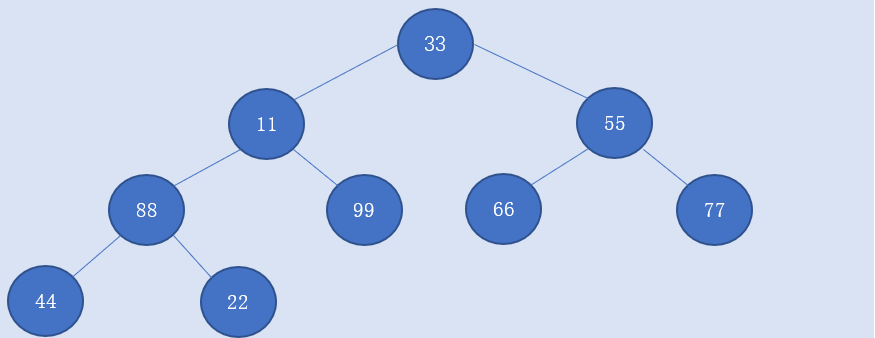2.我们采用小堆排序，将各个子节点和父节点进行比较，若子结点小于父结点，则父结点的元素与子结点的元素交换。以此循环。

3．一轮循环后，根结点的元素即是该序列中的最小值（11）。如图2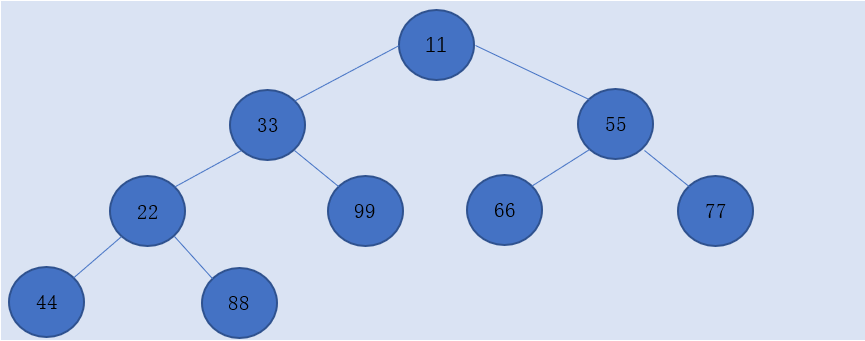4．将根结点和最后一个结点交换，然后之后的计算对最后一个结点不做处理，相当于将这个结点排除在二叉树外（如图3）。用数组实现的二叉树可以认为是将最小值和数组最后一个元素的值交换，然后数组长度减1。但是数组最后一个元素依然存在数组中，只是暂时不对它进行运算处理。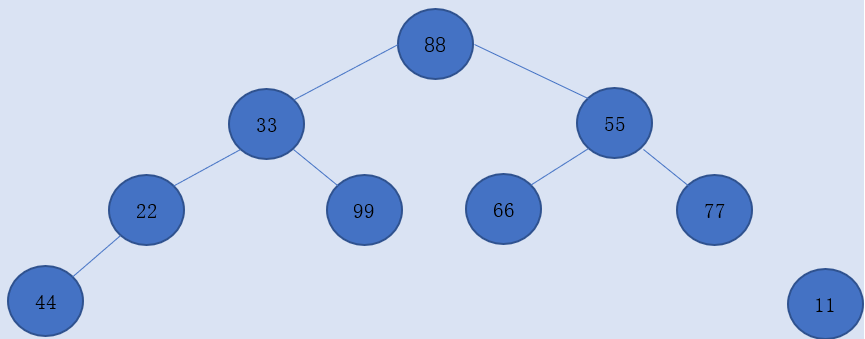5．剩下的元素再进行一次循环交换以及排除元素，结果如图4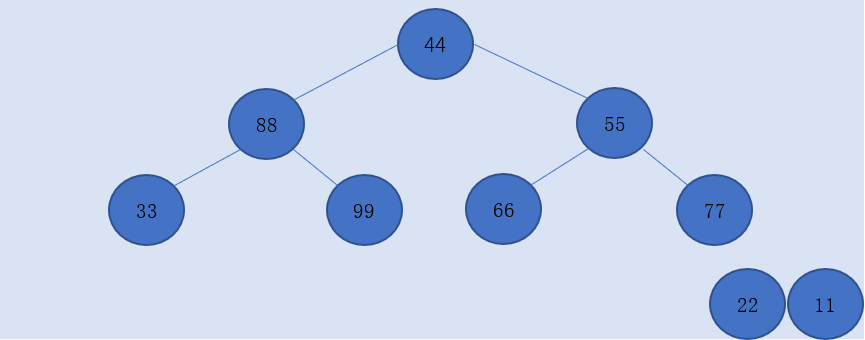6．进行第三次循环，结果如图5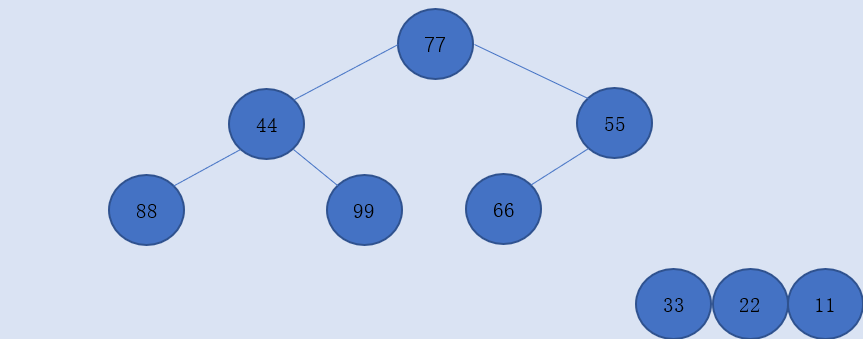7．依次循环，直到只剩下一个根结点，结果如图6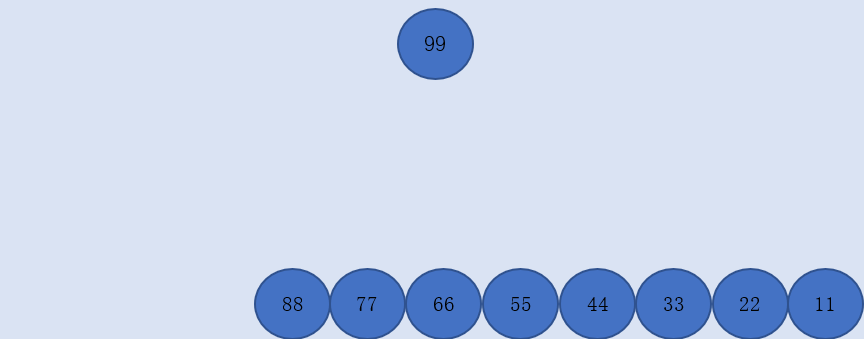8．排序结束，在数组中的结果就是数组的第一个元素到最后一个元素分别是：

99 88 77 66 55 44 33 2211

排序结束后，我们也大值能够总结出堆排序的各项优缺点：

①  堆排序适用于数据量较大的序列排序。对于数据较少的序列来说，堆排序的主要运行时间耗费在创建堆和调整堆上。

②  堆排序的时间复杂度为O(nlogn),他的平均时间、最好时间、最坏时间均为O(nlogn)。

③  不稳定，堆排序会根据相同元素子结点的不同改变各个相同元素的位置。所以不稳定。

④  堆排序的辅助空间只有交换的temp变量，有时甚至temp变量都不需要。

/*****************************************************
File name:Heapsort
Auther name:Kin     Version:1.0    Date: 2018.5.13
*****************************************************/
#include <stdio.h>
#include <stdlib.h>

void Swap(int *a, int *b)//交换函数
{
int temp;
temp = *a;
*a = *b;
*b = temp;
}

void Print(int *a, int length)//打印函数
{
int i;

for (i = 0; i < length; i++)
{
printf("%d ", a[i]);
}
printf("\n");
}

void Minsort(int *a, int parents, int len)
{
int temp;
int child;
for (; parents * 2 + 1 <= len; parents = child)
{
temp = a[parents];
child = 2 * parents + 1;
if (child + 1 <= len && a[child] > a[child + 1])//比较一个父结点的两个子结点的元素大小
{
child = child + 1;
}

if (a[parents] > a[child])                  //比较父结点的元素和两个子结点中较小的子结点元素
{
a[parents] = a[child];
a[child] = temp;
}
}
}

void Heapsort(int *a, int length)//排序函数
{
int i = 0;

for (i = length / 2 -1; i >= 0; i--)//第一轮循环排序
{
Minsort(a, i, length - 1);
}

for (i = length - 1; i > 0; i--)//剩余的循环排序
{
Swap(&a, &a[i]);//交换根结点元素和数组最后一个元素
Minsort(a, 0, i - 1);
}
}

int main(int argc,char **argv)
{
int a[] = {11, 33, 55, 88, 99, 66, 77, 44, 22};//定义为数组
int length = sizeof(a) / sizeof(a);//计算序列长度

Heapsort(a, length);
Print(a, length);

return 0;
}